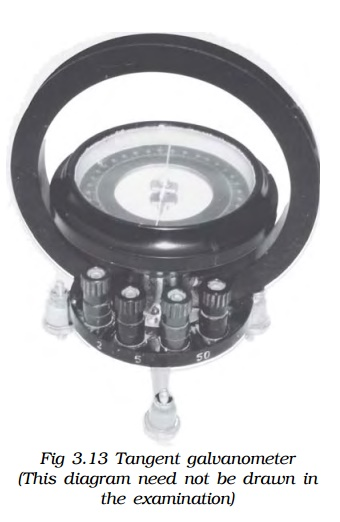Home | | Physics | Biot Savart Law and Magnetic induction

# Biot Savart Law and Magnetic induction

Biot and Savart conducted many experiments to determine the factors on which the magnetic field due to current in a conductor depends.

Biot – Savart Law

Biot and Savart conducted many experiments to determine the factors on which the magnetic field due to current in a conductor depends.The results of the experiments are summarized as Biot-Savart law.

Let us consider a conductor XY carrying a current I (Fig 3.10). AB = dl is a small element of the conductor. P is a point at a distance r from the mid point O of AB. According to Biot and Savart, the magnetic induction dB at P due to the element of length dl is

1.     directly proportional to the current (I)

2.     directly proportional to the length of the element (dl )

3.     directly proportional to the sine of the angle between dl and the line joining element dl and the point P (sin θ)The direction of dB is perpendicular to the plane containing current element Idl and r (i.e plane of the paper) and acts inwards. The unit of magnetic induction is tesla (or) weber m-2.

## 1.Magnetic induction due to infinitely long straight conductor carrying current

XY is an infinitely long straight conductor carrying a current I (Fig 3.11). P is a point at a distance a from the conductor. AB is a small element of length dlθ is the angle between the current element I dl and the line joining the element dl and the point P. According to Biot-Savart law, the magnetic induction at the point P due to the current element Idl is## 2.Magnetic induction along the axis of a circular coil carrying current

Let us consider a circular coil of radius ‘a’ with a current I as shown in Fig 3.12. P is a point along the axis of the coil at a distance from the centre O of the coil.The direction of dB is perpendicular to the current element Idl and CP. It is therefore along PR perpendicular to CP.

Considering the diametrically opposite element AB, the magnitude of dB at P due to this element is the same as that for AB but its direction is along PM. Let the angle between the axis of the coil and the line joining the element (dl) and the point (P) be α.

dB is resolved into two components :- dB sin α along OP and dB cos α perpendicular to OP. dB cos α components due to two opposite elements cancel each other whereas dB sin α components get added up. So, the total magnetic induction at P due to the entire coil is## 3.Tangent galvanometer

Tangent galvanometer is a device used for measuring current. It works on the principle of tangent law. A magnetic needle suspended at a point where there are two crossed fields at right angles to each other will come to rest in the direction of the resultant of the two fields.### Construction

It consists of a circular coil of wire wound over a non magnetic frame of brass or wood. The vertical frame is mounted on a horizontal circular turn table provided with three levelling screws. The vertical frame can be rotated about its vertical diameter.

There is a small upright projection at the centre of the turn table on which a compass box is supported.

The compass box consists of a small pivoted magnet to which a thin long aluminium pointer is fixed at right angles. The aluminium pointer can move over a circular scale graduated in degrees. The scale consists of four quadrants. The compass box is supported such that the centre of the pivoted magnetic needle coincides with the centre of the coil. Since the magnetic field at the centre of the coil is uniform over a very small area, a small magnetic needle is used so that it remains in an uniform field even in deflected position. Usually the coil consists of three sections of 2,5 and 50 turns, which are of different thickness, used for measuring currents of different strength.

### Theory

When the plane of the coil is placed parallel to the horizontal component of Earth’s magnetic induction (Bh) and a current is passed through the coil, there will be two magnetic fields acting perpendicular to each other : (1) the magnetic induction (B) due to the current in the coil acting normal to the plane of the coil and (2) the horizontal component of Earth’s magnetic induction (Bh) (Fig 3.14).Due to these two crossed fields, the pivoted magnetic needle is deflected through an angle θ. According to tangent Law,Since the tangent galvanometer is most sensitive at a deflection of 450, the deflection has to be adjusted to be between 300 and 600.

Study Material, Lecturing Notes, Assignment, Reference, Wiki description explanation, brief detail
Physics : Effects of electric current : Higher Secondary(12 Std) : Biot Savart Law and Magnetic induction |